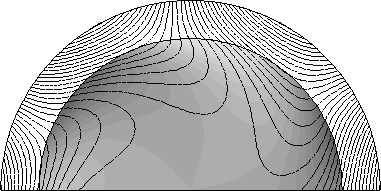QuickField

A new approach to field modelling
 Language: Global English Deutch Espanol Francais Italiano Danmark Ceske Chinese Pycckuü

>> >>

# Conductive cylinder in rotating magnetic field

Problem type:
Plane problem of transient magnetics.

Geometry:

Given:
Conductivity of copper conductor σ = 63 MS/m;
Magnitude of external field B0 = 1 T;
Number of poles 2p = 6;
Frequency f = 50 Hz.

Solution:
To specify rotating magnetic field on the outer boundary of the region, Bn = B0 sin (ωt - pφ), we apply the Dirichlet boundary condition, using the formula:
A = (1/60) * cos (18000*t - 3*atan2 (y/x)).

The coefficient A0 = 1/60 arises from consideration

Bn = (1/r)(∂A/∂φ) = (1/r)*A0p·sin(ωt - pφ)

and A0 = B0·r/p

Due to periodicity of the problem, only half of the model is presented, and odd periodic boundary condition A1 = -A2 is applied on the cut. In fact, it would be enough to simulate just 60° sector of the model. In time domain, problem is simulated with automatic adaptive time step, up to 0.05 seconds (approx. 2.5 periods).

Results:
t = 0.0002 sec:t = 0.048 sec:t = 0.05 sec:• Video
• View simulation report in PDF.
• Download simulation files (files may be viewed using any QuickField Edition).